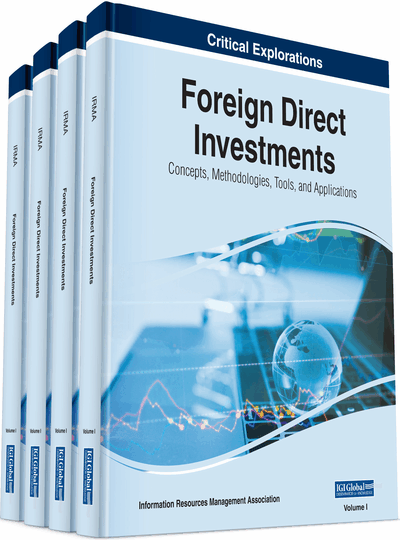# Computational Investing

Javier Vidal-García, Marta Vidal, Rafael Hernandez Barros
DOI: 10.4018/978-1-7998-2448-0.ch002
OnDemand:
(Individual Chapters)
Available
\$37.50
No Current Special Offers

## Abstract

Investing means using funds to start a venture or acquire part of an existing one in hopes that in time the business will accrue profits for the entrepreneur. Often, entrepreneurs opt to purchase companies' stocks. To be able to select the right – profitable – stocks to buy, one requires knowledge of modern portfolio theory, computational investing, stock brokerage, mutual funds, bonds, value investing, how capital gains are taxed, how to trade stocks and options online, and how to use diversification to allocate online investments.
Chapter Preview
Top

## Introduction

The Modern Portfolio Theory is a result of the ingenious efforts of Harry Markowitz. In 1952, Markowitz pioneered the concept by publishing an article, “Portfolio selection,” in the Journal of Finance. Through the piece, he demonstrated how diversification of stock reduces portfolio risk (Strong, 2009, p. 156). In other words, a single investment is exposed to risk due to the fact that several factors create high uncertainty and, as a result, lower expected returns. Examples of such factors are time, resources, manpower, and so on. Thus, Markowitz proposed a model that ensures capital is spread across a variety of investments so as to cushion entrepreneurs against any unfavorable returns resulting from one or several securities.

To gain a better understanding of Modern Portfolio Theory, it is important to understand the key terms applicable in the model. Firstly, risk is the variability in outcomes based on the expected return. Hence, it is measured by calculating the standard deviation (σ) of a percentage of the return (ri). Optionally, risk can be determined by computing variance (σ2), which is the square of standard deviation. Secondly, expected returns (E (ri)) is a function of probability (pi) and percentage of the returns (ri) expected from the security. In this case, the expected returns of a portfolio is a summation of individual returns (∑ pi ri) of several securities. Thirdly, a security includes a finance lease, commercial loan, trade receivable, credit card, and so on. Fourthly, a portfolio is a collection of investment items such as bonds and stocks that require capital allocation. Fifthly, covariance (σij) is a measure of the relationship that exists between variables (Francis and Kim). A positive covariance denotes that the variables progress in a similar direction. In other words, it shows positive correlation. Sixthly, an investment item’s weight (wi) is the ratio of the funds allocated to the stock to the total amount invested in the entire portfolio. Finally, portfolio risk (σp) is a logical expression that is based on the covariance and variance of securities in a portfolio.

Markowitz’s diversification concept is useful in portfolio analysis. In this case, assets that have a positive correlation can be combined with an aim of reducing risk. Hence, that ensures that none of the returns of the portfolio is forfeited. In other words, the risk of the portfolio reaches its lowest value when the correlation between assets is at a minimum (Francis & Doncheol, 2013, p. 5). That remains the case even if the risk associated with individual assets is high.

According to Markowitz, diversification needs to be done strategically. In this case, an entrepreneur should invest in assets in different industries (Strong, 2009, p. 149). For instance, the investor should buy shares in manufacturing, mining, and service sectors. The reason for that is that companies that sell the same goods or services occasionally perform poorly and hence investors that diversify their portfolios by buying assets in the same industry stand to lose. Conversely, by investing in different sectors, poor performance in one industry does not affect the returns from other industries.

The significance of diversification can be illustrated using a portfolio containing two investment assets, A and B. Suppose the expected returns for asset A (E(rA)) is 8%, while that of asset B (E(rB)) is 24%. Also, suppose that the standard deviation for asset A (σA) is 22%, while that of B (σB) is 44%. Additionally, assume that the weight of asset A (wA) is 2/3, while that of asset B (wB) is 1/3. In this case, the expected return of the portfolio (E (rp)) can be determined by the following calculations.

E (rp) = wAE(rA) + wBE(rB)E (rp) = 2/3(8/100) + 1/3(24/100) = 0.0533 + 0.0800E (rp) = 0.1333 = 13.33%

As shown in the example above, the expected return of the portfolio is a weighted average of the expected returns of the assets A and B (Graham & Smart, 2012, p. 219). This means that by investing in two different assets from different industries, the entrepreneur gains favorable returns from the portfolio. Thus, diversification of the portfolio improves the expected returns.

## Complete Chapter List

Search this Book:
Reset